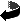# A quadratic lower bound for the number of minimal geodesics by Bernd Ammann, Clara Löh

A quadratic lower bound for the number of minimal geodesics, Preprint version (pdf)

### Abstract

A minimal geodesic on a Riemannian manifold is a geodesic defined on ℝ that lifts to a globally distance minimizing curve on the universal covering. Bangert proved that there is a lower bound for the number of geometrically distinct minimal geodesics of closed Riemannian manifolds that is linear in the first Betti number, using the stable norm unit ball on the first homology. We refine this method to obtain a quadratic lower bound.Back to my Homepage